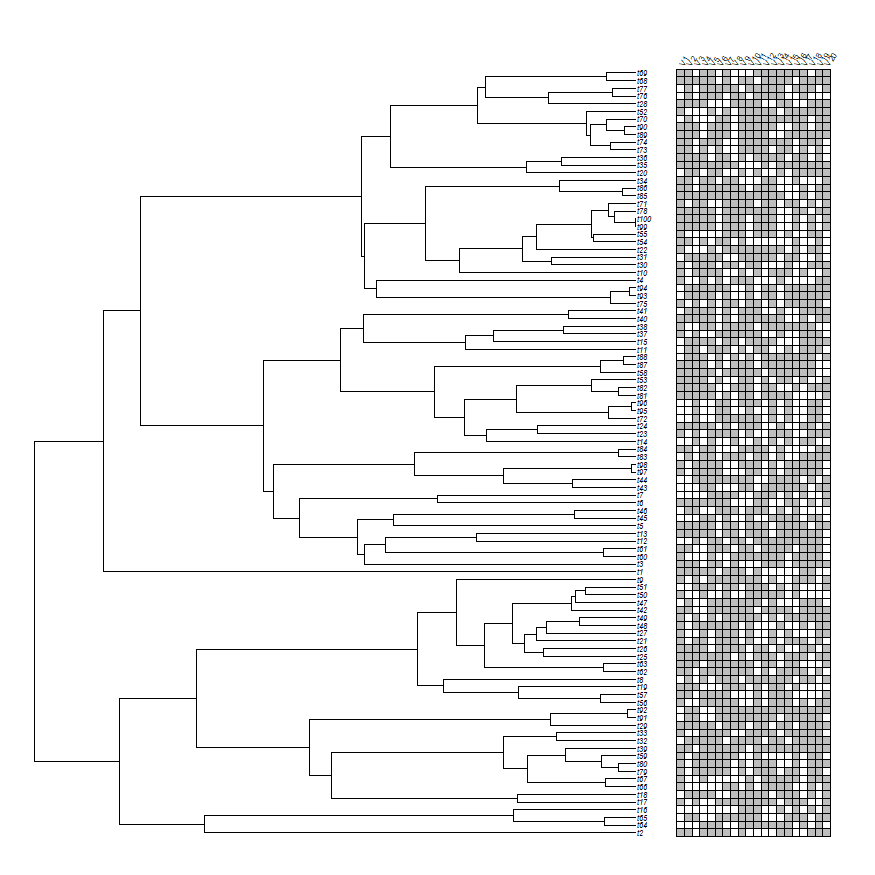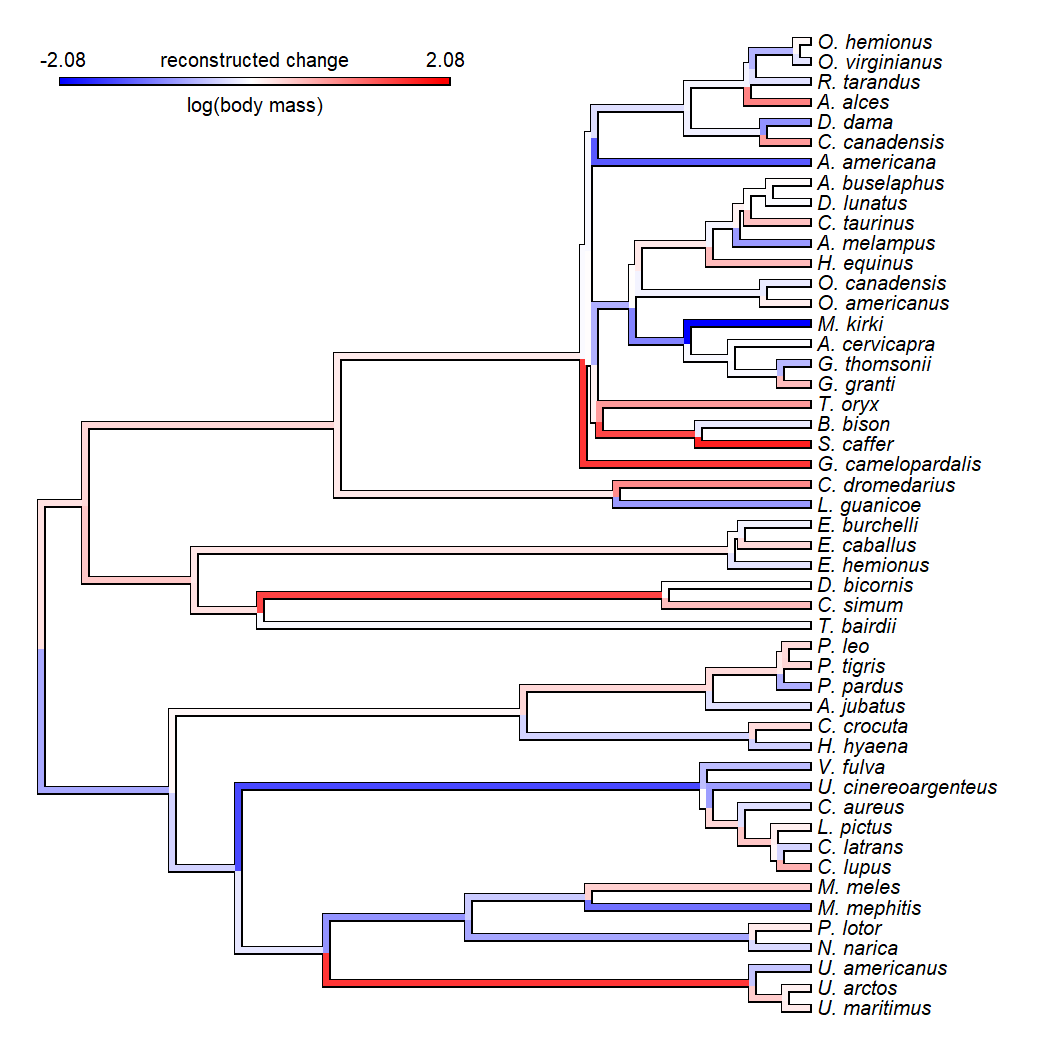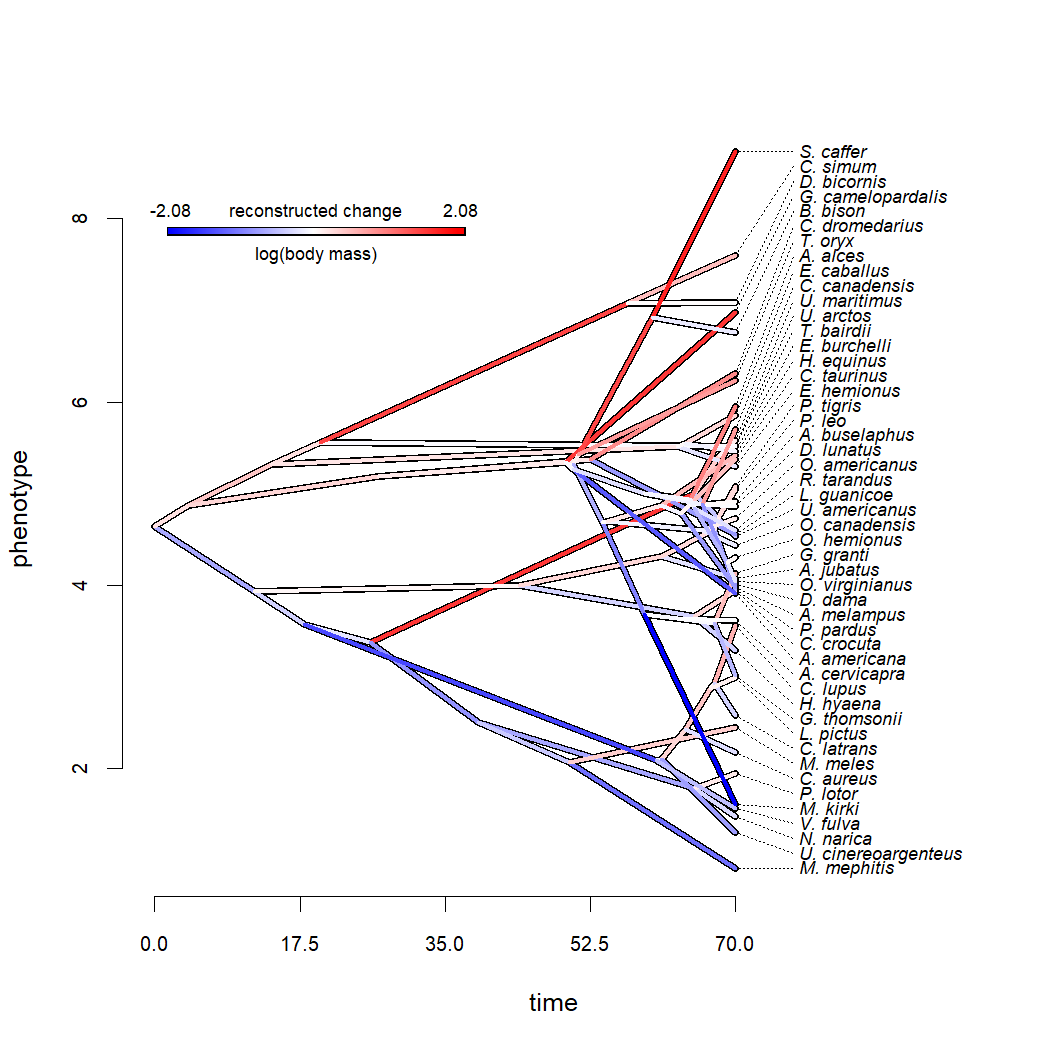## Monday, May 10, 2021

### Fitting a single Mk discrete character evolution model to a set of different discrete traits using likelihood

Recently on the Twitterverse I came across a thread in which the poster described using revBayes to fit an Mk model to multiple discrete traits at the same time, assuming the same value of the transition matrix (Q) for all traits.

This is logical for some traits because fitting the Mk model to a single discrete trait can often be data-limited, particularly if the trait changes infrequently (which will thus leave very little information about the transition process for any particular trait).

This would not make a lot of sense for situations in which, for example, we expected the rate of change to be widely different from trait to trait. On the other hand, it make very good sense if changes are rare, and/or if there are biological reasons to presume that the rate of change in the trait is similar for different traits, and particularly if we intend to proceed to use our estimated value for Q in other analyses, such as in ancestral state reconstruction or stochastic mappping. (More on this in a separate episode!)

Of course, this can also be done in R!

Firstly, it can be done using my phytools function `ratebytree` because this function (by default) fits two models – one in which the transition rates are different across all the trees and a second in which they are all the same.

However, it can also be fit just by making your own custom likelihood function and then optimizing it. I'm going to demonstrate that option here since it will run faster than using `ratebytree` – simply because we're not fitting the multi-rates model too.

First, let me load phytools and then simulate a tree & 20 binary characters on the phylogeny with a constant value of the transition matrix Q as follows.

``````library(phytools)
Q<-matrix(c(-2,1,2,-1),2,2,dimnames=list(0:1,0:1))
Q
``````
``````##    0  1
## 0 -2  2
## 1  1 -1
``````
``````X<-sim.Mk(tree<-pbtree(n=100),Q,nsim=20)
``````
``````##     V1 V2 V3 V4 V5 V6 V7 V8 V9 V10 V11 V12 V13 V14 V15 V16 V17 V18 V19 V20
## t2   1  1  0  1  1  1  1  0  1   0   0   0   0   1   1   0   0   1   1   1
## t64  0  0  0  1  1  1  0  0  1   1   1   1   1   1   1   0   1   0   1   0
## t65  0  1  1  0  0  1  1  1  1   1   1   0   0   0   1   1   1   0   1   1
## t16  0  0  0  0  0  0  0  0  1   1   1   0   0   1   0   1   0   1   0   1
## t17  1  0  1  0  0  1  1  1  1   1   1   1   1   0   1   1   1   0   0   1
## t18  0  1  1  1  1  0  0  1  1   1   1   1   0   1   1   1   0   1   0   1
``````
``````cols<-replicate(20,setNames(c("white","grey"),0:1),
simplify=FALSE)
plotTree.datamatrix(tree,X,sep=0,colors=cols)
``````Readers can see that I simulated data for a binary trait with two levels, 0 and 1, in which the transition rate from 0 → 1 is 2.0; while the transition rate from 1 → 0 is 1.0.

Great, now let's make our likelihood function.

The way I'm going to do this is by using the phytools function `fitMk` but in which I fix the value of Q to a value that I will pre-specify within our likelihood function.

There are other ways we could have done this. For instance, both `fitMk` and `fitDiscrete` in the geiger package also export the likelihood function as part of the model object.

Here's what our likelihood function is going to look like. Note that to use `mapply` to add the log-likelihoods across all the traits, I first recompose my trait data array (`X`) and my Q matrix (`Q`) into lists. In theory this code does not require that I have the same character coding for each trait – merely that I'm willing to assume the same value of Q, given the row/colum order matches the order of the levels of each trait. (It also assumes that each trait has more than one state. It would be a simple fix to relax this assumption.)

``````lik<-function(q,X,tree,trace=0){
X<-lapply(as.list(X),setNames,rownames(X))
Q<-lapply(X,function(x,q)
matrix(c(-q,q,q,-q),2,2,
dimnames=list(levels(x),levels(x))),
q=q)
LIK<-function(x,Q,tree)
-logLik(fitMk(tree,x,fixedQ=Q))
logL<-sum(mapply(LIK,x=X,Q=Q,MoreArgs=list(tree=tree)))
if(trace>0){
cat(paste("q = ",signif(q,5),
"; q = ",signif(q,5),"; logL = ",
signif(-logL,8),"\n",sep=""))
flush.console()
}
logL
}
``````

Now that I have my likelihood function I can go ahead and optimize it using one of R's built-in numerical optimization routines. I will use `optim` – but there are others I could have tried (e.g., `nlminb`).

In `optim` I'll use the method `"L-BFGS-B"` which is a 'box-constrained' routine. Values for Qi,j (for ij) are logically constrained to be ≥ 0, but to avoid problems, I'll set the lower bounds to 10-8. I'll set my upper bound to 100, but this could be adjusted based on the total depth of the tree.

``````fit<-optim(c(1,1),lik,tree=tree,X=X,trace=1,method="L-BFGS-B",
lower=1e-8,upper=100)
``````
``````## q = 1; q = 1; logL = -1278.2533
## q = 1.001; q = 1; logL = -1278.0522
## q = 0.999; q = 1; logL = -1278.4548
## q = 1; q = 1.001; logL = -1278.4422
## q = 1; q = 0.999; logL = -1278.0647
## q = 100; q = 1e-08; logL = -15397.215
## q = 100; q = 1e-08; logL = -15397.215
## q = 99.999; q = 1e-08; logL = -15397.203
## q = 100; q = 0.001; logL = -7820.664
## q = 100; q = 1e-08; logL = -15397.215
## q = 48.459; q = 0.52061; logL = -3109.0536
## q = 48.46; q = 0.52061; logL = -3109.0682
## q = 48.458; q = 0.52061; logL = -3109.039
## q = 48.459; q = 0.52161; logL = -3107.8083
## q = 48.459; q = 0.51961; logL = -3110.3014
## q = 13.116; q = 0.87762; logL = -1927.0841
## q = 13.117; q = 0.87762; logL = -1927.1295
## q = 13.115; q = 0.87762; logL = -1927.0387
## q = 13.116; q = 0.87862; logL = -1926.4874
## q = 13.116; q = 0.87662; logL = -1927.6816
## q = 3.9157; q = 0.97055; logL = -1323.1261
## q = 3.9167; q = 0.97055; logL = -1323.1988
## q = 3.9147; q = 0.97055; logL = -1323.0533
## q = 3.9157; q = 0.97155; logL = -1322.8752
## q = 3.9157; q = 0.96955; logL = -1323.3775
## q = 2.0027; q = 0.98987; logL = -1213.548
## q = 2.0037; q = 0.98987; logL = -1213.5633
## q = 2.0017; q = 0.98987; logL = -1213.5328
## q = 2.0027; q = 0.99087; logL = -1213.5138
## q = 2.0027; q = 0.98887; logL = -1213.5826
## q = 1.9741; q = 1.0321; logL = -1212.2328
## q = 1.9751; q = 1.0321; logL = -1212.2387
## q = 1.9731; q = 1.0321; logL = -1212.2269
## q = 1.9741; q = 1.0331; logL = -1212.2191
## q = 1.9741; q = 1.0311; logL = -1212.2467
## q = 1.9571; q = 1.0611; logL = -1211.9706
## q = 1.9581; q = 1.0611; logL = -1211.9704
## q = 1.9561; q = 1.0611; logL = -1211.9709
## q = 1.9571; q = 1.0621; logL = -1211.9697
## q = 1.9571; q = 1.0601; logL = -1211.9719
## q = 1.9613; q = 1.0652; logL = -1211.9659
## q = 1.9623; q = 1.0652; logL = -1211.9654
## q = 1.9603; q = 1.0652; logL = -1211.9665
## q = 1.9613; q = 1.0662; logL = -1211.9657
## q = 1.9613; q = 1.0642; logL = -1211.9664
## q = 1.9791; q = 1.0764; logL = -1211.9573
## q = 1.9801; q = 1.0764; logL = -1211.9568
## q = 1.9781; q = 1.0764; logL = -1211.9579
## q = 1.9791; q = 1.0774; logL = -1211.958
## q = 1.9791; q = 1.0754; logL = -1211.9569
## q = 1.991; q = 1.0812; logL = -1211.9549
## q = 1.992; q = 1.0812; logL = -1211.9548
## q = 1.99; q = 1.0812; logL = -1211.9551
## q = 1.991; q = 1.0822; logL = -1211.9553
## q = 1.991; q = 1.0802; logL = -1211.9548
## q = 1.993; q = 1.0815; logL = -1211.9548
## q = 1.994; q = 1.0815; logL = -1211.9548
## q = 1.992; q = 1.0815; logL = -1211.9548
## q = 1.993; q = 1.0825; logL = -1211.955
## q = 1.993; q = 1.0805; logL = -1211.9549
## q = 1.993; q = 1.0814; logL = -1211.9548
## q = 1.994; q = 1.0814; logL = -1211.9548
## q = 1.992; q = 1.0814; logL = -1211.9548
## q = 1.993; q = 1.0824; logL = -1211.9549
## q = 1.993; q = 1.0804; logL = -1211.9549
``````
``````fit
``````
``````## \$par
##  1.992985 1.081415
##
## \$value
##  1211.955
##
## \$counts
##       13       13
##
## \$convergence
##  0
##
## \$message
##  "CONVERGENCE: REL_REDUCTION_OF_F <= FACTR*EPSMCH"
``````

Remembering that to simulate our data, we set Q1,2 to be 2.0 and Q2,1 to be 1.0. Our estimates turn out to be really very close.

Now let's compare to `ratebytree` as follows:

``````ratebytree(rep(tree,20),lapply(as.list(X),setNames,rownames(X)),
model="ARD")
``````
``````## ML common-rate model:
##  1->0    0->1    k   logL
## value    1.0812  1.9929  2   -1211.9548
##
## Model fitting method was "optim".
##
## ML multi-rate model:
##  1->0    0->1    k   logL
## tree1    0.6997  1.4017  40  -1200.0165
## tree2    1.007   1.431
## tree3    0.9505  1.6508
## tree4    1.0151  2.0233
## tree5    1.0356  2.6362
## tree6    1.1637  1.7085
## tree7    1.2568  3.7405
## tree8    1.536   2.5116
## tree9    1.3073  2.483
## tree10   0.5318  1.0522
## tree11   1.0557  2.4955
## tree12   0.9176  1.4706
## tree13   1.777   3.7395
## tree14   0.9998  1.6659
## tree15   1.2626  2.1373
## tree16   1.4788  1.9684
## tree17   0.588   1.1634
## tree18   2.4303  4.0818
## tree19   1.0434  2.1037
## tree20   2.4274  4.146
##
## Model fitting method was "nlminb".
##
## Likelihood ratio: 23.8765
## P-value (based on X^2): 0.9641
``````

Neat. This also tells us how varied our fitted model parameter estimated would have been if we fit our Mk model to any of these 20 trait vectors individually!

## Monday, May 3, 2021

### Computing (& plotting) the implied changes from ancestral state reconstruction on the branches of a phylogeny

“I am using phytools to reconstruct ancestral states of a continuous trait on a phylogenetic tree, and was wondering if there is a function in phytools to calculate the number of branches that show an increase in trait value and the number that show a decrease? And if so, if there's a way to visualise this, such as all branches showing an increase in one colour and all showing a decrease in another colour?”

Without advising specifically on whether or not ancestral state reconstructions should be used in this way (I choose to remain agnostic on this point), the answer is, of course 'yes' – this can absolutely be done in R. (To paraphrase Simone Blomberg, with R it's a question of how not if.)

To illustrate this, I will use data for body mass in mammals that come from Garland et al. (1992). Let's load these data, and then estimate ancestral states using `fastAnc`.

``````library(phytools)
data(mammal.tree)
data(mammal.data)
lnMass<-setNames(log(mammal.data\$bodyMass),
rownames(mammal.data))
fit<-fastAnc(mammal.tree,lnMass)
fit
``````
``````## Ancestral character estimates using fastAnc:
##       50       51       52       53       54       55       56       57
## 4.640573 3.941365 3.580030 3.378235 5.008602 5.417042 2.506860 1.783328
##       58       59       60       61       62       63       64       65
## 2.075654 2.092616 2.102696 2.427963 2.879837 2.935833 4.003930 3.660685
##       66       67       68       69       70       71       72       73
## 4.315032 4.607959 4.760302 4.873642 5.317474 5.559303 7.078588 5.517309
##       74       75       76       77       78       79       80       81
## 5.552671 5.192977 5.370860 5.348416 5.312073 5.325223 5.429285 6.920095
##       82       83       84       85       86       87       88       89
## 4.688839 3.685079 3.636850 3.590634 4.698303 4.603683 4.874816 4.790504
##       90       91       92       93       94       95       96       97
## 4.882241 4.886430 5.262581 5.009917 4.889860 4.940759 4.842665 4.247903
``````

Alright. Now, first, how do we simply count the number of edges that involve a recontructed increase in log body mass, compared to those involved an estimated decrease?

This is probably a lot easier than you'd think.

We just need to remember the structure of our `"phylo"` object.

In a `"phylo"` object, the branches of the tree are encoded in a matrix (called `edge`) in which each row contains the starting and ending edge indices of each branch in the phylogeny. If we paste together our original data values (in the order of the tip labels of the phylogeny) and our estimated ancestral states (in the order of increasing node index value), then we'll have a vector containing the observed or reconstructed trait value for each tip and node of the phylogeny, in the order of the node & tip indices. We can then simply restructure this vector into a matrix based on the indices in `edge`.

``````node.vals<-mammal.tree\$edge
node.vals[]<-c(lnMass[mammal.tree\$tip.label],
fit)[mammal.tree\$edge]
``````

Let's look at the first 10 rows of this matrix:

``````head(node.vals,10)
``````
``````##           [,1]     [,2]
##  [1,] 4.640573 3.941365
##  [2,] 3.941365 3.580030
##  [3,] 3.580030 3.378235
##  [4,] 3.378235 5.008602
##  [5,] 5.008602 5.417042
##  [6,] 5.417042 5.579730
##  [7,] 5.417042 5.526647
##  [8,] 5.008602 4.536891
##  [9,] 3.378235 2.506860
## [10,] 2.506860 1.783328
``````

Cool, now let's proceed to simply count the edges over which the trait increased:

``````increases<-sum(node.vals[,2]>node.vals[,1])
increases
``````
``````##  50
``````

This tells is that on 50 edges of the tree, the trait log(body mass) is increasing, according to our reconstruction.

Every (non zero-length) edge must involve an increase or a decrease, so the decreases should be just the number of edges in our tree (96) minus this value (i.e., 46), but let's check just to be sure:

``````decreases<-sum(node.vals[,2]<node.vals[,1])
decreases
``````
``````##  46
``````

The next part of the question was if there is “a way to visualise this, such as all branches showing an increase in one colour and all showing a decrease in another colour”.

Of course we can do this – but I'd say that we can actually do even better.

What I'm going to do is create a custom color gradient such that red is a large positive change; blue is a large negative change; and white is no change at all. Then I'm going to plot the tree with these colors.

Let's see what that looks like:

``````cols<-setNames(
colorRampPalette(c("blue","white","red"))(510),
1:510)
edge.vals<-node.vals[,2]-node.vals[,1]
max.val<-max(abs(edge.vals))+1e-8
intervals<-seq(-max.val,max.val,length.out=511)
intervals<-cbind(intervals[1:510],intervals[2:511])
edge.ind<-sapply(edge.vals, function(x,intervals)
intersect(which(x>intervals[,1]),which(x<=intervals[,2])),
intervals=intervals)
painted<-paintBranches(mammal.tree,mammal.tree\$edge[1,2],
state=edge.ind)
for(i in 2:length(edge.ind))
painted<-paintBranches(painted,mammal.tree\$edge[i,2],
state=edge.ind[i])
plot(painted,colors=cols,lwd=4,split.vertical=TRUE,
outline=TRUE,ftype="i",fsize=0.8)
title="reconstructed change",subtitle="log(body mass)",
lims=c(-max.val,max.val),digits=2,prompt=FALSE,x=2,
y=Ntip(mammal.tree)-2,fsize=0.8)
``````Pretty cool.

For fun, let's take a look at how this appears on a traitgram. Edges going up (that is, towards increasing values for the trait) should be in red, edges going down should be in blue.

Let's check.

``````par(cex.axis=0.8)
lwd=5)
``````
``````## Optimizing the positions of the tip labels...
``````
``````par(fg="transparent")
``````## Optimizing the positions of the tip labels...
``````par(fg="black")